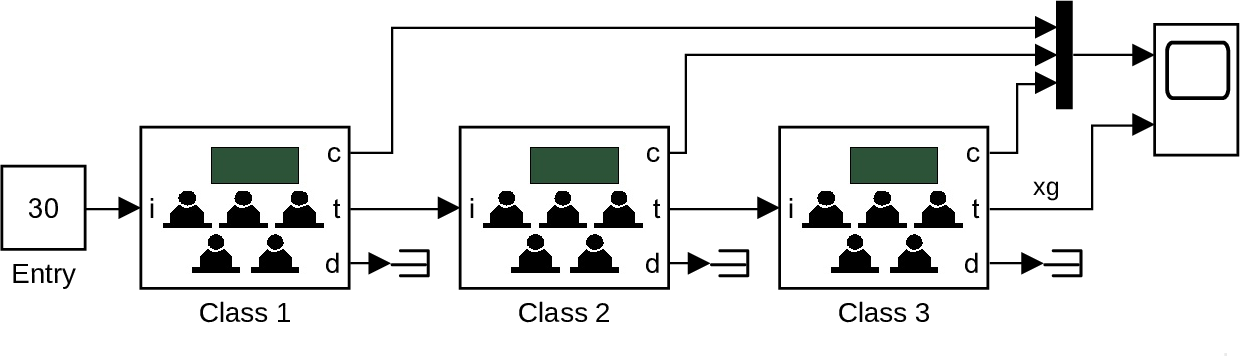### Straightforward implementation

• Simulink implementation of Version A:
• completely standard
• define subcomponent for a class
•• uses UnitDelay to store xi(k)
• complete model
•• Implementation of Version B:
• create subsystem Binomial for random numbers ξ
• ξ ~ B(n, p), p as parameter, n as input
• easy with Matlab function
• new class component
•